# High-Low Method

Used in the field of management accounting, which is an essential part of accounting.

To understand the high-low method, first, we need to understand management accounting. The high-low method is used in the field of management accounting, which is an essential part of accounting.Management accounting refers to identifying, analyzing, and communicating financial information to a firm's managers to achieve the company's future goals.

Management accounting involves decision-making, planning, coordinating, controlling, communicating, and motivating. Similar to management accounting and financial accounting, there is cost accounting to determine the cost of a product.

Management accounting involves the past, present, and future of a business with systematic recording, reporting, and analysis of financial transactions (accuracy, cost-effectiveness, critical analysis).

Management accountants work for public companies, private companies, and government offices. Their role is to collect, observe, and record numbers; advise on the company's investments and manage them; budgeting, planning, risk management, and decision-making.

Similar to management accounting, cost accounting is the process of allocating costs to cost items, which often comprise a business's products, services, and other activities. Cost accounting is useful because it can show where a company spends money, how much it earns, and where it loses money.

Cost accounting is used for several purposes, such as standard costing, activity-based costing, lean accounting, and marginal costing.

Calculating fixed and variable costs is a part of cost accounting. Fixed costs are monthly expenses that do not change depending on the level of production. Rent, depreciation, interest on loans, and lease charges are all examples.

Cost management allows us to forecast future expenses and plan accordingly. It also aids in the control of project costs and the pre-determination of maintenance costs. We can examine long-term company trends and achieve the business goals with proper cost management.

## What are costs & cost behavior?

A cost is an expense needed to sell, create or acquire assets for a product or service. In other words, it is the monetary value of expenditure for supplies, services, etc. For example, if the cost of a liter of milk is \$2, the consumer has to spend \$2 to acquire a liter of milk.Cost accounting is a type of managerial accounting that attempts to capture a company's entire cost of production by analyzing both variable and fixed costs, such as a leasing fee. They are generated internally by the company's managers.

It is commonly practiced to assist managers in making crucial business decisions, as it provides them with actual statistics and critical data that help with decisions.

Cost accounting also helps in minimizing product costs as it highlights the reports of profit. Apart from these, it is a part of making sustainability efforts.

Costs consist of four aspects:

• Elements are used for cost control (whether they relate to material, labor, or expense).

• Function (labor, material, production cost, non-production cost, administration cost, distribution cost).

• Nature (how they relate to production) (direct and indirect costs).

• Behavior (how they change in response to levels of output or activity), (fixed, variable, and so on).

Cost behavior describes how costs change as a result of changes in business activities. For example, the electricity cost for a firm will increase when working hours are increased.

They differ in how they change as a result of changes in various business activities such as increased or decreased production, plans of expansion, budgeting for the firm, investing, etc.

## Types of cost behavior

Costs are further differentiated into various sub-parts, which have their own significance in accounting and economics. The cost subdivision is discussed as follows:• Fixed costs - These are also known as period costs, overhead costs, or supplementary costs. These are the expenses that are not dependent on the level of business activity, but the fixed cost per unit decreases as activity increases. It is calculated by dividing fixed costs into units.

The formula for fixed costs is -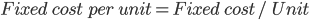• Semi-Fixed/stepped costs - These are costs that are constant for a given level of activity but increase or decrease when the standard threshold is crossed. They are not proportional to the business activity levels.

Some common examples of these costs are supervision costs and marketing costs.

• Variable cost - This is known as the cost per unit, prime, and direct costs. This cost is directly proportional to the number of units and thus has an effect on volume changes; it is expressed as total variable costs per activity level.

Variable costs are volume related. This means that they are dependent on the business activity.

Variable cost is the product of cost per unit and the total number of units. Some common examples of these are raw materials, labor, delivery costs, production supplies, and packaging supplies. The formula for Variable cost is: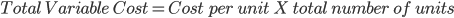Relevant/ Irrelevant costs - These are also known as avoidable and unavoidable costs. Avoidable costs are the ones that are affected by the decision of a manager, whereas unavoidable costs are costs that are not affected by the decision of managers.

An example of a relevant cost is future cost and opportunity cost, whereas irrelevant cost is sunk cost and committed cost.

• Sunk cost - These are also known as retrospective costs. These are the costs that are created by past decisions and cannot be changed by a decision in the future. Examples include salaries, marketing, research, facilities expenses, etc.

It can be calculated by subtracting the present realizable salvage value from the book value. For example, buying 2,000 shares of company A at \$10 a share, for instance, represents a sunk cost of \$20,000.

• Opportunity Cost - This is the cost that measures the opportunity that is lost when a choice of a course requires another to give up. An example is someone who gives up going to see a movie to study for a test in order to get a good grade. The opportunity cost is the cost of the movie and the enjoyment of seeing it.

## Semi-variable cost

This is the cost that features the high-low method for its calculation. This cost includes a fixed charge and a variable element (fixed cost + variable element). Semi-variable costs are neither constant in total nor per unit.However, to identify these costs, we need to observe the cost behaviors strongly.

The cost behaviors that are to be observed for each cost are:

Fixed Cost - These costs are always constant per unit.

Variable Cost - These are also constant per unit.

Semi-Variable Cost - These expenses are not constant in total or per unit.

Stepped Cost - These costs are fixed in total.

When the fixed cost in a semi-variable cost is less than the break-even point (when the company makes profit = loss), it is considered good. This cost is relevant when predictions are made on a company's financial performance across different production lines.

A method to calculate semi-variable costs is the Least Squares Regression method, or linear method of calculation, which is formulated as: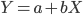Where Y = Total Semi-Variable Cost, a = Fixed Cost, b = Variable Cost per unit, and X = the number of units produced. The observations made from this method are not accurate.

This method has disadvantages in that it fits a straight line to any set of cost data, regardless of how unpredictable the cost behavior pattern is. Furthermore, unless you have access to a computer, computations necessitated by the least squares approach are tedious and time-consuming.

Therefore, the observations made from this method are not accurate. There are other methods, such as the analytical approach and the scatter graph method, but the high-low method is considered the most convenient.

## The high-low method

This is also known as the "high low points" method. It is used to calculate the semi-variable cost. This method looks at the entire cost difference between two volumes and divides the extra cost by the volume.

• The two levels of volume chosen are the maximum and lowest during the periods under consideration, as the words "high" and "low" suggest. The projected variable cost per unit is the outcome of this division.The average activity level and the average cost for the periods in the database are then computed. The fixed cost is calculated by subtracting the variable cost for the average activity level from the total average cost.

Formulas to remember are -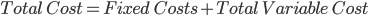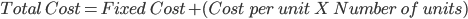To learn the high-low method, we will be taking an example -

Production UnitsTotal Cost
10,000150,000
12,000170,000
14,000190,000
15,000200,000

Step 1 -

Pick the highest and lowest levels of activity in the set of data and their associated costs.

Highest level of production = 15,000 units costing \$200,000

Lowest level of production = 10,000 units costing \$150,000

Change = 5,000 units and \$50,000

Step 2 -

Calculate the variable cost per unit using the following formula and the activity levels identified in step one:

Variable cost per unit = Change in cost ÷ change in units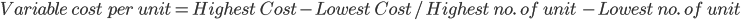To calculate the total fixed cost,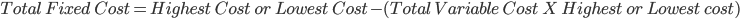Step 3 -

Pick either the highest or lowest level of activity and fill in what we know.

Using 10,000 units:

Total cost = Total fixed cost + Total variable cost

\$150,000 = Total fixed cost + (\$10 × 10,000 units)

Therefore total fixed costs = \$50,000

Step 4 -

Apply to a new level of activity

For 17,000 units:

Total cost = fixed cost + (cost per unit × number of units)

Total cost = \$50,000 + (\$10 × 17,000 units)

Total cost = \$50,000 + \$170,000

Total cost (forecast) = \$220,000

The high-low method is easy to use, understand, and quick to work around. No complex set of tools or programs is required for it to function. Although this is a really easy and understandable method, there are a few shortcomings to this method that make it less practical.

We should be really careful when choosing the data for calculation with this tool, as any small mistake can lead to an inaccurate result.

The reason for this is quite simple: cost can be affected by various economic elements and cannot be accurately predicted with just two variables.

After a certain level of production, a firm requires more fixed investments, which cannot be covered by this method; therefore, this method should be used with extreme caution.

Limitations of this method are -

• The method's first flaw is that it presumes a linear relationship between cost and activity, which might not be the case.

• It simply assumes two activity levels, and as a result of that, it doesn't reflect the complete data set correctly.

• This method does not account for changes in fixed and variable costs over time.

## Key Takeaways

• Managerial Accounting is a vital part of accounting and involves decision-making, planning, coordinating, controlling, communicating, and motivating.
• Cost accounting is the process of allocating costs to cost items, which often comprise a business's products, services, and other activities.
• Cost accounting is used for several purposes, such as standard costing, activity-based costing, lean accounting, and marginal costing.
• Cost is the monetary value of expenditure for supplies and services. It includes four aspects - element, nature, function, and behavior.
• Costs are further divided based on their behavior into fixed costs, variable costs, semi-fixed costs, and semi-variable costs.
• The high-low method is used in calculating semi-variable costs, and It compares the highest and lowest activity levels, as well as the costs associated with each level.
• The two levels of volume chosen are the maximum and lowest during the periods under consideration, as the words "high" and "low" suggest while calculating.
• Stepwise calculation with an example is discussed in the article.
• With all the benefits this method provides, there are some drawbacks, such as not accounting for changes in fixed and variable costs over time.
• The high-low method is easy to use, understand, and quick to work around. No complex set of tools or programs is required for it to function.
• To work with difficult methods of finance, such as the high-low method, individuals need to build a strong foundation in finance. WallStreetOasis provides its members with a course in finance for students as well as professionals.

Researched and authored by Abhinav BhardwajLinkedIn